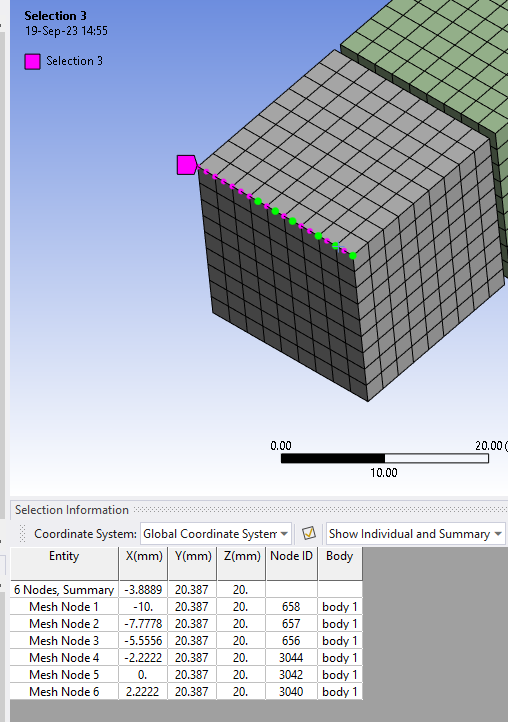# How to plot stress results on specific coordinates in Mechanical?Member Posts: 2Hello,

Is it possible to plot the stress values of my model at certain coordinates (theoretical weld transition) in Mechanical? The coordinates result from the joint lines of two plates and a defined evaluation distance to the joint lines. Thus, they usually do not lie exactly on a node, but can also lie in the middle of an element. So some kind of mapping is probably necessary.
The result should look similar to a path stress result, which graphically displays values relatively independent of the underlying meshing.

The coordinates and stress components at the evaluation points have already been calculated. The question now is how I can manage a graphical output.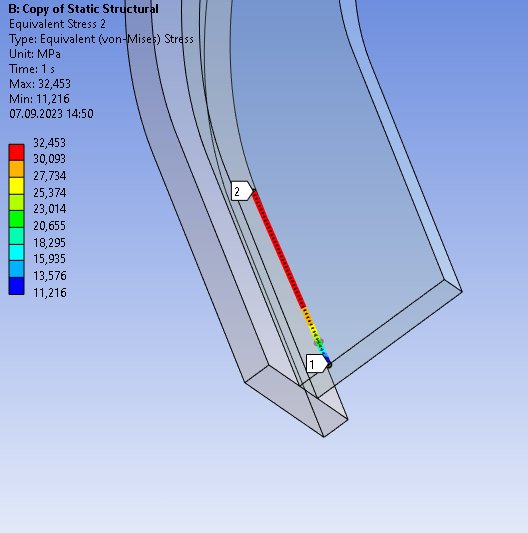Tagged:

•Member, Moderator, Employee Posts: 535Hello @Philipp_92 , if you're in Mechanical I would recommend creating a Python Result and use DPF to map the results. You can refer to this post that should help: https://discuss.ansys.com/discussion/2418/python-result-example-mapping-data

Also, there's an example in the PyDPF documentation that would be helpful:
https://dpf.docs.pyansys.com/version/0.8/examples/06-plotting/04-plot_on_path.html#sphx-glr-examples-06-plotting-04-plot-on-path-py
You'll have to adapt it to Mechanical DPF but the code would be mostly the same.

•Member Posts: 2Hello @Pernelle Marone-Hitz ,

I have created a equivalent stress result for defined nodes and now trying to get the same result using a python result with a defined coordinate field.

Below the equivalent stress result from ansys and the node coordinates: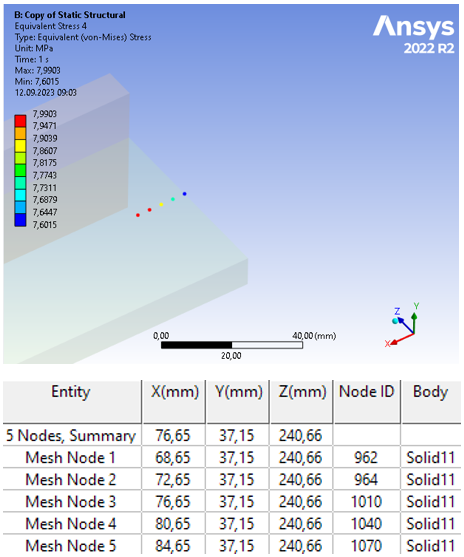I created a coordinate field from the node coordinates and now trying to do the equivalent stress mapping on those points. Apparently the mapping works, but the graphical output gives the values at the wrong positions.

My recent script looks like this:

```def define_dpf_workflow(analysis):

import mech_dpf
import Ans.DataProcessing as dpf
import Ans.DataProcessing.operators as ops
mech_dpf.setExtAPI(ExtAPI)
dataSource = dpf.DataSources(analysis.ResultFileName)

s = ops.result.stress_von_mises()
s.inputs.data_sources.Connect(dataSource)

coords = [68.65,37.15,240.66]
delta = 4
n_points = 5
for i in range(1,n_points):
x_offs = 1
y_offs = 0
z_offs = 0
initialX = coords
tempX = initialX+x_offs*delta*i
initialY = coords
tempY = initialY+y_offs*delta*i
initialZ = coords
tempZ = initialZ+z_offs*delta*i
coords.append(tempX)
coords.append(tempY)
coords.append(tempZ)

field_coord = dpf.FieldsFactory.Create3DVectorField(numEntities=len(coords))
field_coord.Data = coords
field_coord.ScopingIds = list(range(1,len(coords)/3+1))

model = dpf.Model(analysis.ResultFileName)
mesh = model.Mesh
mop = ops.mapping.on_coordinates()
mop.inputs.fields_container.Connect(s)
mop.inputs.coordinates.Connect(field_coord)
mop.inputs.create_support.Connect(True)
mop.inputs.mesh.Connect(mesh)

output = ops.utility.forward()
output.inputs.any.Connect(mop)

dpf_workflow = dpf.Workflow()
dpf_workflow.SetOutputContour(output)
dpf_workflow.Record('wf_id', False)
this.WorkflowId = dpf_workflow.GetRecordedId()
```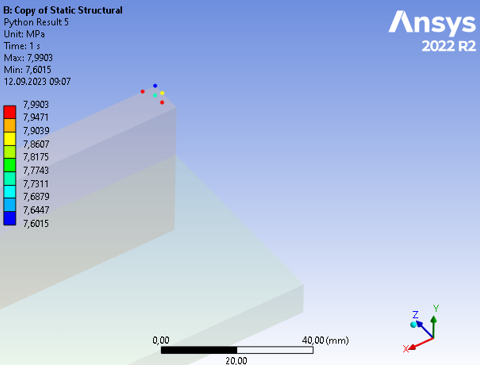looks like the mapped stresses are plotted to the scoping ids from the coordinates field, which contains the nodes [1,2,3,4,5] from the model.

•Member, Moderator, Employee Posts: 535Hi @Philipp_92 , sorry for the late answer, I was out of office. I had a quick look at your code and I think there might be an issue with the new mesh that you are creating to map the results. In the PyDPF example, coordinates are a list of list: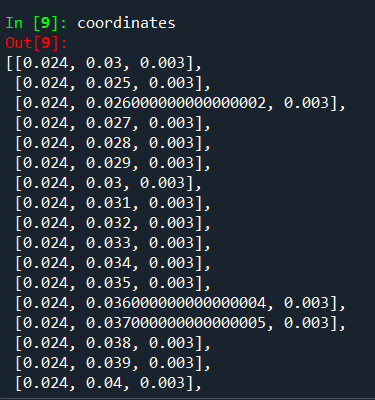and we can see that these position vary along the Y axis.
In your example, coords seems to be a single list: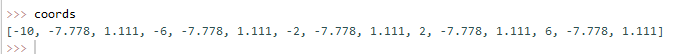and the way the values vary is unclear.
In that list, the values seem off (I used my own set of values to match the dummy model I'm using). Nodal position of some nodes in the named selection: